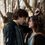# Start at Basel Problem

In 18th century, Euler solved a well known problem, even Leibniz cannot solve it, either. This event made him become famous and confident. Basel problem shows $$\displaystyle \lim_{n \to +\infty} \left(\dfrac{1}{1^2}+\dfrac{1}{2^2}+\dfrac{1}{3^2}+\cdots+\dfrac{1}{n^2}\right)= \sum_{m=1}^{\infty} \dfrac{1}{m^2}=\dfrac{\pi^2}{6}$$. It is a wonderful theory, but I’m not satisfied with this. We can use it to find more interesting conclusions. \begin{align*} \because \dfrac{\pi^2}{6} &=\dfrac{1}{1^2}+\dfrac{1}{2^2}+\dfrac{1}{3^2}+\cdots \\ \therefore \dfrac{1}{2^2} \times \dfrac{\pi^2}{6} &=\dfrac{1}{2^2} \times \dfrac{1}{1^2}+\dfrac{1}{2^2} \times \dfrac{1}{2^2}+\dfrac{1}{2^2} \times \dfrac{1}{3^2}+\cdots \\ \therefore \dfrac{1}{2^2} \times \dfrac{\pi^2}{6} &=\dfrac{1}{2^2}+\dfrac{1}{4^2}+\dfrac{1}{6^2}+\cdots \\ \therefore \left(1-\dfrac{1}{2^2}\right) \dfrac{\pi^2}{6} &=\dfrac{1}{1^2}+\dfrac{1}{3^2}+\dfrac{1}{5^2}+\cdots \\ \therefore \dfrac{\pi^2}{8} &=\dfrac{1}{1^2}+\dfrac{1}{3^2}+\dfrac{1}{5^2}+\cdots \\ \dfrac{\pi^2}{24} &=\dfrac{1}{2^2}+\dfrac{1}{4^2}+\dfrac{1}{6^2}+\cdots \\ \therefore \left(\dfrac{1}{8}-\dfrac{1}{24}\right)\pi^2 &=\dfrac{1}{1^2}-\dfrac{1}{2^2}+\dfrac{1}{3^2}-\cdots \\ \therefore \dfrac{\pi^2}{12} &=\dfrac{1}{1^2}-\dfrac{1}{2^2}+\dfrac{1}{3^2}-\cdots \\ \end{align*} It’s true that Basel problem has an important relationship with Riemann $$\zeta$$ function, because Basel problem is in order to find $$\zeta(2)$$. About 100 years later, Riemann used one equation to build a bridge between functions and prime numbers. The equation is $$\displaystyle \zeta(s) =\sum_{n=1} ^{\infty} \dfrac{1}{n^s}= \prod_{p \text{ prime}} ^{\infty} (1-p^{-s})^{-1}$$. Here is Euler's proof. \begin{align*} \because \zeta(s) &=\dfrac{1}{1^s}+\dfrac{1}{2^s}+\dfrac{1}{3^s}+\cdots \\ \therefore \dfrac{1}{2^s} \zeta(s) &=\dfrac{1}{2^s}+\dfrac{1}{4^s}+\dfrac{1}{6^s}+\cdots \\ \therefore \left(1-\dfrac{1}{2^s}\right)\zeta(s) &=\dfrac{1}{1^s}+\dfrac{1}{3^s}+\dfrac{1}{5^s}+\cdots \\ \because \dfrac{1}{3^s} \times \left(1-\dfrac{1}{2^s}\right)\zeta(s) &=\dfrac{1}{3^s} \times \dfrac{1}{1^s}+\dfrac{1}{3^s} \times \dfrac{1}{3^s}+\dfrac{1}{3^s} \times \dfrac{1}{5^s}+\cdots \\ \therefore \left(1- \dfrac{1}{3^s}\right) \left(1-\dfrac{1}{2^s}\right)\zeta(s) &=\dfrac{1}{1^s}+\dfrac{1}{5^s}+\dfrac{1}{7^s}+\cdots \\ \therefore \left(1-\dfrac{1}{5^s}\right)\left(1- \dfrac{1}{3^s}\right)\left(1-\dfrac{1}{2^s}\right)\zeta(s) &=\dfrac{1}{1^s}+\dfrac{1}{7^s}+\dfrac{1}{11^s}+\cdots \\ \therefore 1 &=\zeta(s)\left(1-\dfrac{1}{2^s}\right)\left(1- \dfrac{1}{3^s}\right)\left(1-\dfrac{1}{5^s}\right)\cdots \\ \therefore \zeta(s) &=\dfrac{1}{\left(1-\dfrac{1}{2^s}\right)\left(1- \dfrac{1}{3^s}\right)\left(1-\dfrac{1}{5^s}\right)\left(1-\dfrac{1}{7^s}\right)\cdots} \\ \therefore \sum_{n=1} ^{\infty} \dfrac{1}{n^s} &= \prod_{p \text{ prime}} ^{\infty} (1-p^{-s})^{-1} \end{align*}Note by Edward Christian
1 year, 5 months ago

This discussion board is a place to discuss our Daily Challenges and the math and science related to those challenges. Explanations are more than just a solution — they should explain the steps and thinking strategies that you used to obtain the solution. Comments should further the discussion of math and science.

When posting on Brilliant:

• Use the emojis to react to an explanation, whether you're congratulating a job well done , or just really confused .
• Ask specific questions about the challenge or the steps in somebody's explanation. Well-posed questions can add a lot to the discussion, but posting "I don't understand!" doesn't help anyone.
• Try to contribute something new to the discussion, whether it is an extension, generalization or other idea related to the challenge.
• Stay on topic — we're all here to learn more about math and science, not to hear about your favorite get-rich-quick scheme or current world events.

MarkdownAppears as
*italics* or _italics_ italics
**bold** or __bold__ bold
- bulleted- list
• bulleted
• list
1. numbered2. list
1. numbered
2. list
Note: you must add a full line of space before and after lists for them to show up correctly
paragraph 1paragraph 2

paragraph 1

paragraph 2

[example link](https://brilliant.org)example link
> This is a quote
This is a quote
    # I indented these lines
# 4 spaces, and now they show
# up as a code block.

print "hello world"
# I indented these lines
# 4 spaces, and now they show
# up as a code block.

print "hello world"
MathAppears as
Remember to wrap math in $$ ... $$ or $ ... $ to ensure proper formatting.
2 \times 3 $2 \times 3$
2^{34} $2^{34}$
a_{i-1} $a_{i-1}$
\frac{2}{3} $\frac{2}{3}$
\sqrt{2} $\sqrt{2}$
\sum_{i=1}^3 $\sum_{i=1}^3$
\sin \theta $\sin \theta$
\boxed{123} $\boxed{123}$

## Comments

Sort by:

Top Newest

Log in to reply

Are you actually 13?

- 1 year, 1 month ago

Log in to reply

My friend, you’re just 12, too.

- 10 months, 3 weeks ago

Log in to reply

×

Problem Loading...

Note Loading...

Set Loading...